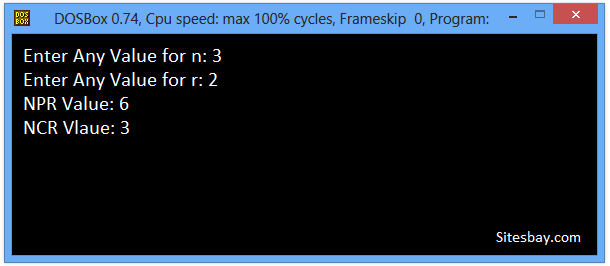# C++ Program Find ncR and nPr of any Number

## C++ Program to Find ncR and nPr of any Number

Basically in NCR, AB is a single combination. But in NPR, AB and BA are two different permutations of a combination of A and B. The difference is quite clear and is easy for us to note that permutations are always equal to of greater in most cases than the combinations of elements. Also note that any number raised to the power of zero is one. This is a situation encountered during calculation of NCR and NPR.

To understand below example, you have must knowledge of following C++ programming topics; For Loop in C++, Function C++ and Function Arguments in C++

## Find ncR and nPr of any Number in C++

```#include<iostream.h>
#include<conio.h>

long int fact(int);   //function declaration
void main()
{
clrscr();
int n, r;
long int ncr, npr;
cout<<"Enter Any Value for n: ";
cin>>n;
cout<<"Enter Any Value for r: ";
cin>>r;
npr=fact(n)/fact(n-r);    // function calling
ncr=npr/fact(r);          //function calling
cout<<"NPR value = "<<npr<<"\n";
cout<<"NCR value = "<<ncr<<"\n";
getch();
}
long int fact(int x)          //defining the function
{
int i, f=1;
for(i=2; i<=x; i++)
{
f=f*i;
}
return f;
}

```

## Output

```Enter Any Value for n: 3
Enter Any Value for r: 2
NPR Value: 6
NCR Vlaue: 3
```## Pure VPN Privide Lowest Price VPN Just @ \$1.65. Per Month with Non Detected IP Lowest Price Non Detected IP VPN